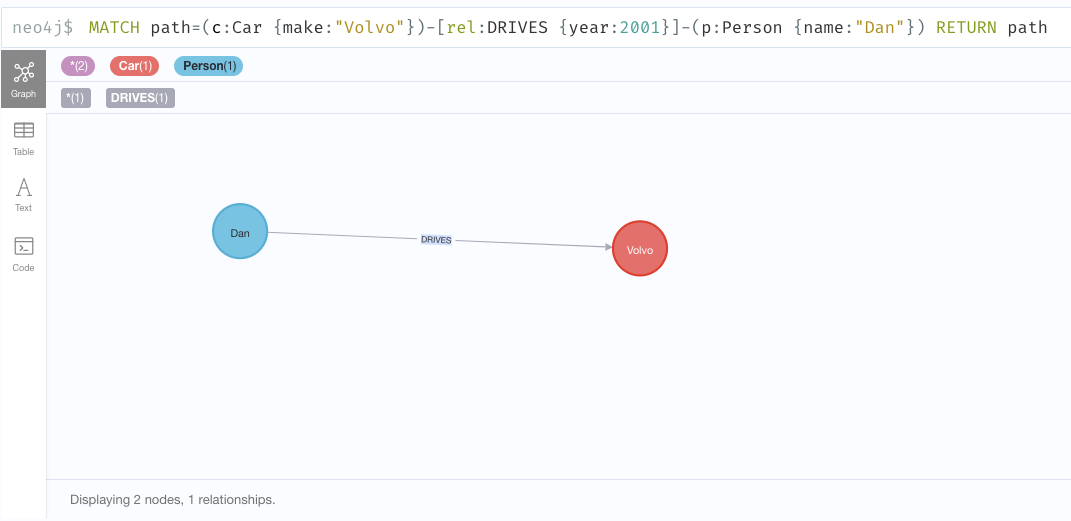# Invert relationships

The APOC library contains a procedure that can be used to invert the direction of relationships.

## Procedure for inverting the direction of relationships

Qualified Name Type

`apoc.refactor.invert(rel RELATIONSHIP)` - inverts the direction of the given `RELATIONSHIP`.

Procedure

## Example

The below example will further explain this procedure.

The following creates a graph containing two nodes connected by a relationship:
``CREATE path=(c:Car {make:"Volvo"})-[rel:DRIVES {year:2001}]->(p:Person {name:"Dan"}) RETURN path``The following inverts the direction of the relationship:
``````MATCH (c:Car)-[rel:DRIVES]->(p:Person)
CALL apoc.refactor.invert(rel)
yield input, output
RETURN input, output``````
Table 1. Results
input output

2

{"identity":3,"start":9,"end":8,"type":"DRIVES","properties":{"year":2001}}

The relationship has now been inverted
`````` ----
MATCH path=(c:Car {make:"Volvo"})-[rel:DRIVES {year:2001}]-(p:Person {name:"Dan"}) RETURN path
----``````